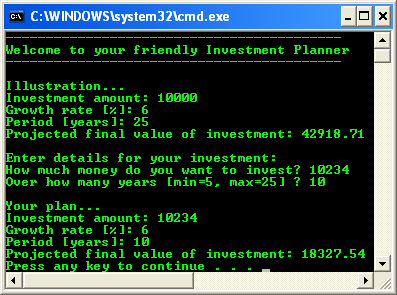# C++ .NET Functions 2

The following are the topics available in this part.

6. # Calling Functions in the Sample Application

Defining Function Bodies

In the previous section, you learned how to declare function prototypes. As you saw, a function prototype specifies the name of a function, its parameter list, and its return type. However, function prototypes do not contain any executable statements; they do not indicate what the function will do when it is called. To provide the behavior for a function, you must define a function body. The function body contains executable statements to perform the desired operations in the function. In this section, you will define function bodies for all the function prototypes introduced earlier.

Defining a Simple Function Body

The following example shows a simple function body, corresponding to the DisplayWelcome function prototype you saw earlier:

void DisplayWelcome()

{

Console::WriteLine(L"------------------------------------------");

Console::WriteLine(L"Welcome to your friendly Investment Planner");

Console::WriteLine(L"------------------------------------------");

return;

}

Notice that the first line of the function body is identical to the function prototype, except that there is no semicolon. This first line is known as the function header.

After the function header, a pair of braces ({}) encloses the executable statements for the function body. In this example, the DisplayWelcome function displays a simple welcome message on the screen. In the next two sections you’ll see more complex functions that perform console input and mathematical calculations.

The return keyword at the end of the function causes flow of control to return to the calling function. In this example, the return keyword is superfluous because the closing brace of the function acts as an implicit return. However, you can use return in other locations in a function, such as within an if statement, to return prematurely from a function. In this exercise, you will add the DisplayWelcome function body to your Visual C++ application.

Continue working with the project you created earlier in this module.

Locate the end of the main() function. On the next line, define the DisplayWelcome function body as follows:

void DisplayWelcome()

{

Console::WriteLine(L"------------------------------------------");

Console::WriteLine(L"Welcome to your friendly Investment Planner");

Console::WriteLine(L"------------------------------------------");

return;

}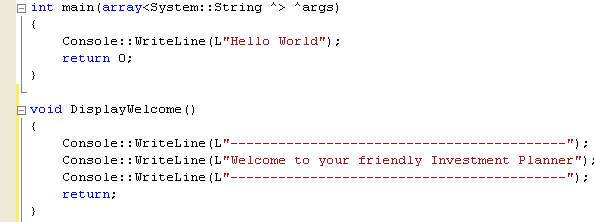Build your program. You shouldn’t get any compiler errors.

You can define function bodies in any order in Visual C++. For example, you can place the DisplayWelcome function body before or after the main() function body. However, functions cannot be nested. You can’t define one function body inside the braces ({}) of another function.

Defining a Function Body That Uses Parameters

When you define a function body that uses parameters, you must define exactly the same number and types of parameters as in the function prototype. This is quite reasonable; the whole point of the function prototype is to introduce the exact signature of the function.

The function body can use different parameter names than the prototype because the parameter names in the prototype are there just for documentation. However, for consistency, you should use the same parameter names in the prototype and the function body.

In this exercise, you will define a function body for the DisplayProjectedValue function. You saw the prototype for this function earlier.

void DisplayProjectedValue(double amount, int years, double rate);

The function body will have the same signature as the prototype and will calculate the projected value of an investment after a specified number of years at a particular growth rate.

Continue working with the project from the previous exercise.

Scroll to the end of the program, and add the following code. This is the start of the DisplayProjectedValue function body:

void DisplayProjectedValue(double amount, int years, double rate)

{

Next define some local variables within the function:

double rateFraction = 1 + (rate/100);

double finalAmount = amount * Math::Pow(rateFraction, years);

finalAmount = Math::Round(finalAmount, 2);

Here the rateFraction variable holds the growth rate as a fractional value. For example, if the rate is 6 percent, rateFraction will be 1.06. The expression Math::Pow(rateFraction, years) shows how to raise a number to a power in Visual C++. For example, Math::Pow(1.06, 3) is equivalent to 1.06 * 1.06 * 1.06. The expression Math::Round(finalAmount, 2) rounds finalAmount to two decimal places. For example, if finalAmount is 1000.775, the rounded value will be 1000.78.

Now add the following statements to the function to display the result of the calculations:

Console::Write(L"Investment amount: ");

Console::WriteLine(amount);

Console::Write(L"Growth rate [%]: ");

Console::WriteLine(rate);

Console::Write(L"Period [years]: ");

Console::WriteLine(years);

Console::Write(L"Projected final value of investment: ");

Console::WriteLine(finalAmount);

return;

}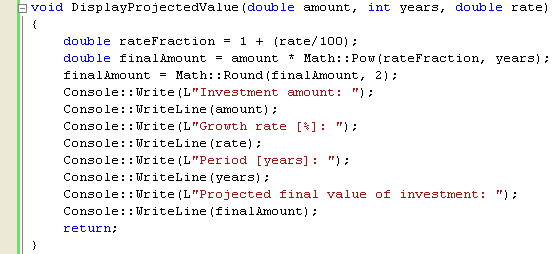Build your program. Make sure there is no error.

Defining a Function Body That Returns a Value

When you define a function with a non-void return type, you must return an appropriate value from the function. To return a value, use the return keyword followed by the value you want to return. If you forget to return a value, you’ll get a compiler error on the closing brace of the function. This point is where the compiler realizes you haven’t returned a value from the function.

In this exercise, you will define a function body for the GetInvestmentAmount function. Here is the prototype for the function, as you saw earlier:

double GetInvestmentAmount();

The function will ask the user how much money he wants to invest. The function will return this value as a double data type. You will also define a function body for the GetInvestmentPeriod function. The prototype for this function is as follows:

int GetInvestmentPeriod(int min = 10, int max = 25);

The function will ask the user how long he wants to invest the money and will return this value as an int data type.

Continue working with the project from the previous exercise.

Scroll to the end of the program, and define the GetInvestmentAmount function body as follows:

double GetInvestmentAmount()

{

Console::Write(L"How much money do you want to invest? ");

double amount = Convert::ToDouble(input);

return amount;

}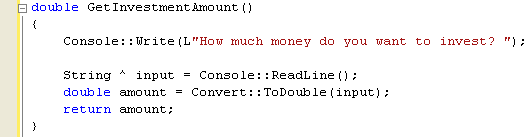The first statement displays a prompt message on the console, asking the user how much money she wants to invest. The Console::ReadLine function call reads a line of text from the console keyboard, and the result is assigned to a String variable. The Convert::ToDouble(input) function call parses the String and converts it to a double value. The return statement returns this value back to the calling function. You can declare local variables anywhere in a function. For example, here the input and amount variables are declared halfway down the GetInvestmentAmount function. Typically, you should declare variables at the point where they are first needed in the function, which is different from the C programming language, where you have to declare local variables at the start of a block.

Now add the following function body just after the GetInvestmentAmount:

int GetInvestmentPeriod(int min, int max)

{

Console::Write(L"Over how many years [");

Console::Write(L"min=");

Console::Write(min);

Console::Write(L", max=");

Console::Write(max);

Console::Write(L"] ? ");

int years = Convert::ToInt32(input);

return years;

}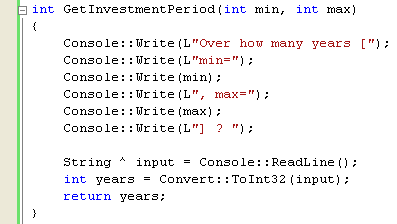The Console::Write function calls ask the user to enter a value between min and max; these values are supplied as parameters into the GetInvestmentPeriod function. The Console::ReadLine function call reads the user’s input as a String, and the Convert::ToInt32(input) function call converts this value into a 32-bit integer. The return statement returns this value to the calling function. The function prototype for GetInvestmentPeriod declared default values for the min and max parameters. The default value for min is 10, and the default value for max is 25. Default values are specified only in the function prototype, you don’t mention these default values in the function body. If you accidentally define the default values in the function body as well as in the function prototype, you’ll get a compiler error at the function body.

Calling Functions

Now that you have defined all the function bodies in the sample application, the last step is to call the functions at the appropriate place in the application.

To call a function, specify its name followed by a pair of parentheses. For example, you can call the DisplayWelcome function as follows:

DisplayWelcome();

This is a simple example because the function doesn’t take any parameters or return a value. If you want to call a function that returns a value, you can assign the return value to a variable. The following example calls the GetInvestmentAmount function and assigns the return value (a double) to a local variable named sum:

double sum = GetInvestmentAmount();

You can ignore the return value from a function if you want. When you call the function, leave out the assignment operator on the left side of the function name. The function still returns the value, but the value is discarded. If you want to call a function that takes parameters, pass the parameter values between the parentheses in the function call. The following example calls the DisplayProjectedValue function, passing in three literal values as parameters:

DisplayProjectedValue(10000, 25, 6.0);

You don’t specify the parameter data types when you call a function. Just provide the parameter values. The following example shows how to call a function that takes parameters and returns a value. In this example, we call the GetInvestmentPeriod function to get a value between 5 and 25. We assign the return value to a local int variable named period:

int period = GetInvestmentPeriod(5, 25);

Calling Functions in the Sample Application

In this exercise, you will extend your sample application to include the function calls you’ve just seen.

Continue working with the project from the previous exercise. Locate the main() function. Just after the opening brace ({) of the main() function (replacing the Hello World Console::WriteLine), add the following statement, which calls the DisplayWelcome function:

DisplayWelcome();

Next add the following statements to display an illustration of investment growth. The DisplayProjectedValue function call will display the value of 10,000 after 25 years at a growth rate of 6 percent.

Console::WriteLine(L"\nIllustration...");

DisplayProjectedValue(10000, 25, 6.0);

Next add the following statements to ask the user how much she wants to invest and for how long. The GetInvestmentAmount and GetInvestmentPeriod function calls return these values.

double sum = GetInvestmentAmount();

int period = GetInvestmentPeriod(5, 25);

The GetInvestmentPeriod function has default values for each of its parameters. The first parameter has a default value of 10, and the second parameter has a default value of 25. You can use these default values when you call the function. For example, the following function call uses the default value for the second parameter:

// First parameter is 5; second parameter defaults to 25

int period = GetInvestmentPeriod(5);

If you use a default value for a parameter, you must use the default values for each subsequent parameter in the parameter list. For example, the following function call is invalid:

// Try to use default value for just the first parameter – illegal.

int period = GetInvestmentPeriod(, 20);

Now add the following statements to calculate and display the projected final value of this investment, assuming a growth rate of 6 percent:

DisplayProjectedValue(sum, period, 6.0);Build your program, and fix any compiler errors. Now run the program, and you should see output similar to the following screen snapshot.